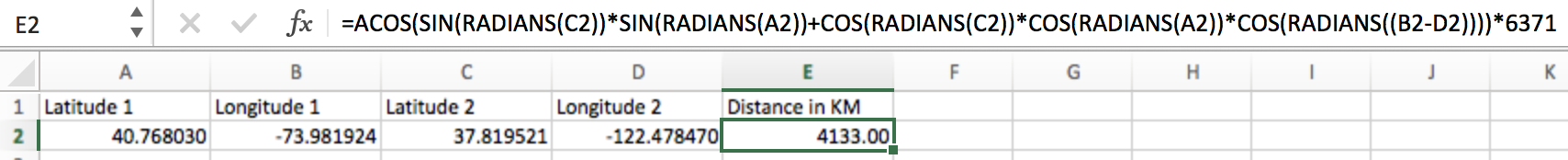# Tutorial: Distance between coordinates

The coordinates returned from the Geolake API are a latitude and longitude in degrees in decimal form. The latitude is 0 at the Equator and goes to +90 at the North Pole and -90 at the South Pole. The longitude is 0 at Greenwich, England and goes down to the west to -180 and up to the east to +180.

If you know the latitude and longitude for two points, you can calculate the distance between them. The easiest method to calculate this distance assumes that the earth is a perfect sphere, which is not entirely true. The results will not be entirely correct, but they will be a reasonable approximation in many cases.

Precise distance calculation is more complicated, and depends on more accurate models of the earth. It's usually more convenient to use an existing library for precise distance calculation.

## Rough Approximation

`1 degree of latitude ~= 69 miles (111 km), regardless of the longitude`
`1 degree of longitude ~= 69 miles (111 km) at the Equator where latitude = 0, but this varies by latitude`
```Per latitude: Latitude +10 or -10, 1 degree of longitude = 68 miles (109km) Latitude +20 or -20, 1 degree of longitude = 65 miles (104km) Latitude +30 or -30, 1 degree of longitude = 60 miles (96km) Latitude +40 or -40, 1 degree of longitude = 53 miles (85km) Latitude +50 or -50, 1 degree of longitude = 44 miles (71km) Latitude +60 or -60, 1 degree of longitude = 35 miles (56km) Latitude +70 or -70, 1 degree of longitude = 24 miles (38km) Latitude +80 or -80, 1 degree of longitude = 12 miles (19km) Latitude +90 or -90, 1 degree of longitude = 0 miles/km```

## Excel distance approximationA2 = Latitude 1
B2 = Longitude 1
C2 = Latitude 2
D2 = Longitude 2

Formula for distance in km:
=ACOS(SIN(RADIANS(C2))*SIN(RADIANS(A2))+COS(RADIANS(C2))*COS(RADIANS(A2))*COS(RADIANS((B2-D2))))*6371

Replace 6371 at the end with 3958.76 to get a distance in miles instead

## Java distance approximation

``````double earthRadius = 6371;// Approximate radius of the earth in kilometers

/**
* Get the distance in kilometers between two coordinates.
* @param lat1 Latitude of the first coordinate, in degrees
* @param long1 Longitude of the first coordinate, in degrees
* @param lat2 Latitude of the second coordinate, in degrees
* @param long2 Longitude of the second coordinate, in degrees
* @return Distance in kilometers
*/
public double getDistance(double lat1, double long1, double lat2, double long2) {
double distance = Math.acos(Math.sin(lat2 * Math.PI / 180.0) * Math.sin(lat1 * Math.PI / 180.0) +
Math.cos(lat2 * Math.PI / 180.0) * Math.cos(lat1 * Math.PI / 180.0) *
Math.cos((long1 - long2) * Math.PI / 180.0)) * earthRadius;
return distance;
}``````

## Java precise distance

This example uses https://geographiclib.sourceforge.io/

Maven library dependency

``````<dependency>
<groupId>net.sf.geographiclib</groupId>
<artifactId>GeographicLib-Java</artifactId>
<version>1.49</version>
</dependency>``````

Code

``````import net.sf.geographiclib.Geodesic;
import net.sf.geographiclib.GeodesicLine;
import net.sf.geographiclib.GeodesicMask;

private static Geodesic geod = Geodesic.WGS84;// This matches EPSG4326, which is the coordinate system used by Geolake

/**
* Get the distance between two points in meters.
* @param lat1 First point's latitude
* @param lon1 First point's longitude
* @param lat2 Second point's latitude
* @param lon2 Second point's longitude
* @return Distance between the first and the second point in meters
*/
public static double getDistance(double lat1, double lon1, double lat2, double lon2) {
GeodesicLine line = geod.InverseLine(lat1, lon1, lat2, lon2, GeodesicMask.DISTANCE_IN | GeodesicMask.LATITUDE | GeodesicMask.LONGITUDE);
return line.Distance();
}``````